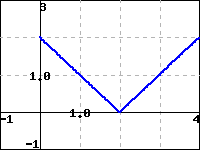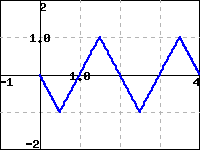The functions $f(t)$ and $g(t)$ are shown below.$f(t)$ $g(t)$

If the motion of a particle whose position at time $t$ is given by $x=f(t)$, $y=g(t)$, sketch a graph of the resulting motion and use your graph to answer the following questions:

(a) The slope of the graph at $\left(1.75,-0.5\right)$ is
(enter undef if the slope is not defined)

(b) At this point the particle is moving and .

(c) The slope of the graph at $\left(0.25,-0.5\right)$ is
(enter undef if the slope is not defined)

(d) At this point the particle is moving and .